Mass, Length, and Topology
19th May 2019
HOME

"There are at present fundamental problems in theoretical physics awaiting solution, e.g., the relativistic formulation of quantum mechanics and the nature of atomic nuclei (to be followed by more difficult ones such as the problem of life), the solution of which problems will presumably require a more drastic revision of our fundamental concepts than any that have gone before."

- P.A.M. Dirac; Proc. Roy. Soc. A 133, 60 1931.

" In any case, models must, of course, be constructed in accordance with acceptable physical theory since the values which distinguish the cosmological speculations of the scientist from those of the crank arise from  the attempt of the former to make his work logical and coherent with the rest of physics."

- Richard Chance  Tolman; Relativity, Thermodynamics, and Cosmology.

"Neither space nor time has any existence outside the system of evolving relationships that comprises the universe.  Physicists refer to this feature of general relativity as background independence."

- Lee Smolin; Three Roads to Quantum Gravity.

Previously we introduced a constant jML with dimensions of Mass-Length, for the topology in the discrete measurement space, defined as: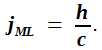One would expect a dimensionless constant for a true topological space.  However we are developing a description for the discrete measurement space or j-space, in which the topology is defined as the entropy-free space.  The entropy-free space implies that time will not be a factor.  Thus the constant jML, is time-independent.  (Topology represents the path connectivity in measurement space, we will discuss it in a later blog.)

We then discussed Compton scattering and introduced Compton wavelength λCompton  which is independent of the energy of the photon being scattered, and hence in our context jML once expressed in terms of λCompton , is independent of the measurement apparatus.  The constant jML should have the same value for elementary particles as well as macroscopic structures such as black holes.  Therefore if we calculated: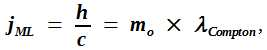where mo is the rest mass for elementary particles, the value of jML should come out to be the same for every elementary particle.  The following table shows the calculated value of jML for some of the elementary particles1:

 Particle Rest mass mo Compton wavelength λCompton Calculated value of jML Electron 9.109 382 91 × 10-31 2.426 310 2389 × 10-12 2.210 218 902 × 10-42 Muon 1.883 531 475 × 10-28 11.734 441 03 × 10-15 2.210 218 902 × 10-42 Tau 3.167 47 × 10-27 0.697 787 × 10-15 2.210 219 389 × 10-42

No surprises here, as λCompton's are calculated values to begin with.  It is just a sanity check confirming that in the relativistic region, which represents the underlying topological structure for a discrete measurement space, the value of the time-independent constant jML, does not change.

Here we will like to point out that, it is a standard practice in relativistic physics to assume h = 1 and c = 1.  However in a discrete measurement space we place the ratio h/c equal to one, instead of h = 1 and c = 1 individually 2.  (h÷c = 1 leads to Mass × Length = 1 or M  = Length-1, the length in this case would represent
λCompton.)

Now the fun part!  Let us consider next a relativistic structure observed at the cosmic scale, a black hole.  The Schwarszchild metric in General Theory of Relativity, is given as: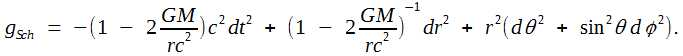We move into the topological space by making the metric gSch time independent, i.e. we equate the coefficient of  dt2 to zero3.  This gives us, Schwarszchild radius as,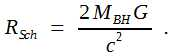RSch is the radius defining the event-horizon of a black hole, and MBH is the mass of the black hole.  We can define the corresponding wavelength as,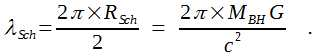Subsequently using the criterion that the constant jML, representing the inherent topology of a given measurement space, does not change for all the structures in j-space, we can write,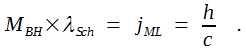Substituting for λSch in above expression, we obtain the expression for Planck mass,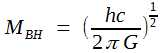.

The estimated mass of a black-hole is of the order of solar mass, approximately 2 × 1030 kg.  In topological space, this mass is equivalent to Planck mass, 2.18 × 10-8 kg.  The difference is truly extraordinary.  So what does the mass really represent?  Is the value of the measured mass an intrinsic property or is it observer dependent?  Let us first review the information in hand.

1. Planck mass corresponding to RSch is measured for the least energy surface surrounding the topological structure.  The actual black hole mass in units of solar mass is measured in the metric space or the reference frame of a macroscopic observer ObsM, where the least energy surface acts as the definition of the origin.  The actual information content of both measurements remains the same, yet mass measured, differ by many orders of magnitude.

2. Aku and his Bubble represent the macroscopic observer ObsM, who measures MBH in units of solar mass.  Let us represent RSch in Kruskal-Szekeres coordinates as shown below.  In this representation, we do not have to worry about the geometrical singularity at the origin 3.  The Aku's measurements estimating MBH are in the exterior region-I.  The Planck mass MP lies in the interior region-II.  So how do we reconcile two very different values for the measurements of mass of same topological object?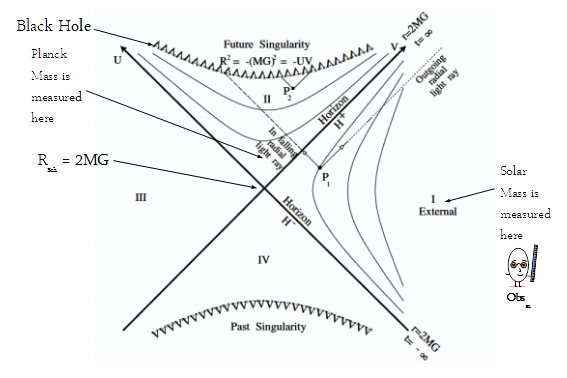If we recall, RSch represents the value for black hole dimensions, below which the inner mechanism of the black hole become important.  This is also the region where Euclidean geometry used to describe the region-I, is no longer applicable.  Aku or ObsM has fairly limited capability (v << c).  Assuming that Aku proceeds into the black hole to make required measurements, and if his progress is monitored by another observer in the region-I, per this observer it will take Aku forever to enter the black hole.  Thus it is impossible for an observer in the region-I who is characterizing the black hole in terms of physical quantities such as temperature, mass, angular momentum, and lifetime etc., to precisely measure the insides of the black hole.

The entropy of black hole measurement is very high, due to limitations of the electron-photon interaction (q = 3 space) based measurement apparatus available to Aku.  Even if Aku was inside RSch, the amount of information which needs to be measured is enormous and consequently in all likelihood Aku will continue to move towards the black hole without successfully completing the required measurements.

Let us review the relationship between G and MBH , corresponding to the topology of the interior region-II,.

In order to move to the topology in the exterior region-I, we can replace MBH with the Zero-Entropy mass mZE , measured by the maximum efficiency observer Obsc.  Recall that the zero-entropy observer means the condition ds2 = 0j, where ds is the space-time interval given as ds2 = gμν dsμdsν, and therefore mass mZE  corresponds to the condition for the space-time interval equal to zero (0j).  The relationship becomes,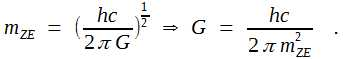We note that on the right hand side we have universal constants h and c, along with zero-entropy mass mZE .  Since the mass mZE   is measured by Obsc, it will also act as an universal constant for Aku's (equivalently ObsM's) measurements in exterior region-I.  Therefore in exterior region-I, G  is expressed in terms constants h, c, and mZE , each of which is a universal constant for the exterior region-I, and hence itself is a universal constant for the exterior region-I (only), denoted by GI.  Note that GI is not GNewton, the known universal constant of gravitation.  (Note: Knot-Theory describes exterior region-1.)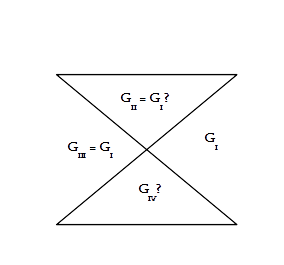Similarly to derive the relationship for G in the region-II, we replace mZE with mp , and use the relationship GII =  hc÷(2πmp2), where mp is Planck mass.  At this point we can not be sure that mZE   and mp are equal, unless they can be precisely measured.  (More importantly the value of G in the region-IV GIV , can not assumed to be equal to GII .)

What if there was another observer, Obsi from higher information space4, whose measurement instruments are much more advanced?  Obsi is able to measure all the information inside the black hole in one measurement, i.e. Obsi is able to perform a zero-entropy measurement same as Obsc, plus Obsi has higher capabilities.  Then we can ask, what would be the nature of the black hole for Obsi, a shallow-well (just another inconvenient pot-hole) or more formidable infinite-potential well (actual black hole)?  In other words, would a black hole in q = 3 information space, exist as a black hole in q = 2 or q = 1 information spaces?  One thing for sure, the observer from q = 3 information space, definitely does not want to know about black holes in q = 2 or q = 1 information spaces.

The black hole exists in Aku's reference frame, because the topology of the information space can not be measured with precision.  Thus a very high measured value of mass corresponding to an infinitesimal information content δi, is specific to the exterior region-I5.  If that is the case, then high value of mass measured in region-I for a specific information content, is an intrinsic property specific to the entropy of the region-I only.  We already know that the entropy of region-I is an invariant, in fact the entropy is the only invariant in the relativistic thermodynamic description of region-I.  Then what is the relationship between the entropy of the measurement and the mass?  Do we have to introduce information-mass besides inertial and gravitational masses?  We will discuss this rather fascinating topic some other time.  Meanwhile perhaps we can speculate on the actual mass of the universe itself and how to measure it with absolute precision?

___________________

1.  Based on the values for Compton wavelengths from National Institute of Standards and Technology (NIST).  The units are in MKS.
2.  The condition of h/c = 1 rather than h = 1 and c = 1 raises an interesting scenario.  In the measurement space of a macroscopic observer, entropy and hence corresponding time-axis are bound to exist.  However it is also plausible that values of the fundamental constants would also change.  Since these constants relate to Planck space, a macroscopic observer need to perform experiments at the edge of the universe, to detect variations in their values.  If such measurements were possible, the constants h and c, are likely to show similar variations, variations which are not independent of each other.
3.  In discrete measurement space, we are not interested in the removal of the geometrical singularity at the origin.  It is understood that a certain region smaller than the Least Energy Surface, will remain inaccessible to Aku and hence could not be measured by Aku.  We note that the Least Energy Surface provides with the definition of origin 0j in discrete measurement space.  More discussion can be found here

We will also like to point out that by making metric gSch time independent, the coefficient of the dr2 term, (~|c2/0j|), becomes extremely large.  This term in not measurable.  Subsequently the effect of variation in a time independent metric corresponding to the topological space δi, will show up as variations in dθ2 and dφ2, i.e. rotational components only, with a massive singularity accompanying dr2 term in Aku's measurement space.  No surprises here as we already know that internal symmetries do show up as various angular momenta in measurements.
4.  We remember that the electron-photon interaction corresponds to q = 3 space.  The higher information spaces are either q = 1 or q = 2.
5.  The infinitesimal information content δi, is the only true invariant in all four regions of space-time in Kruskal-Szekeres coordinates.

***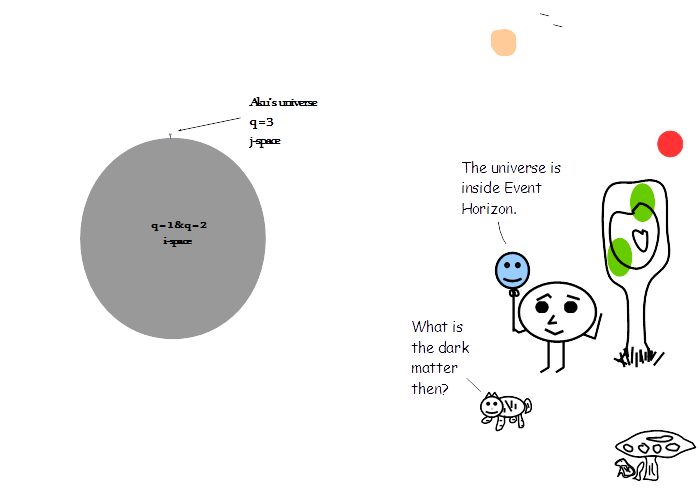Previous Blogs:

EPR Paradox-I
Nutshell-2015

Information on www.ijspace.org is licensed under a Creative Commons Attribution 4.0 International License.
Attribution — You must give appropriate credit, provide a link to the license, and indicate if changes were made. You may do so in any reasonable manner, but not in any way that suggests the licensor endorses you or your use. No additional restrictions — You may not apply legal terms or technological measures that legally restrict others from doing anything the license permits. This is a human-readable summary of (and not a substitute for) the license.